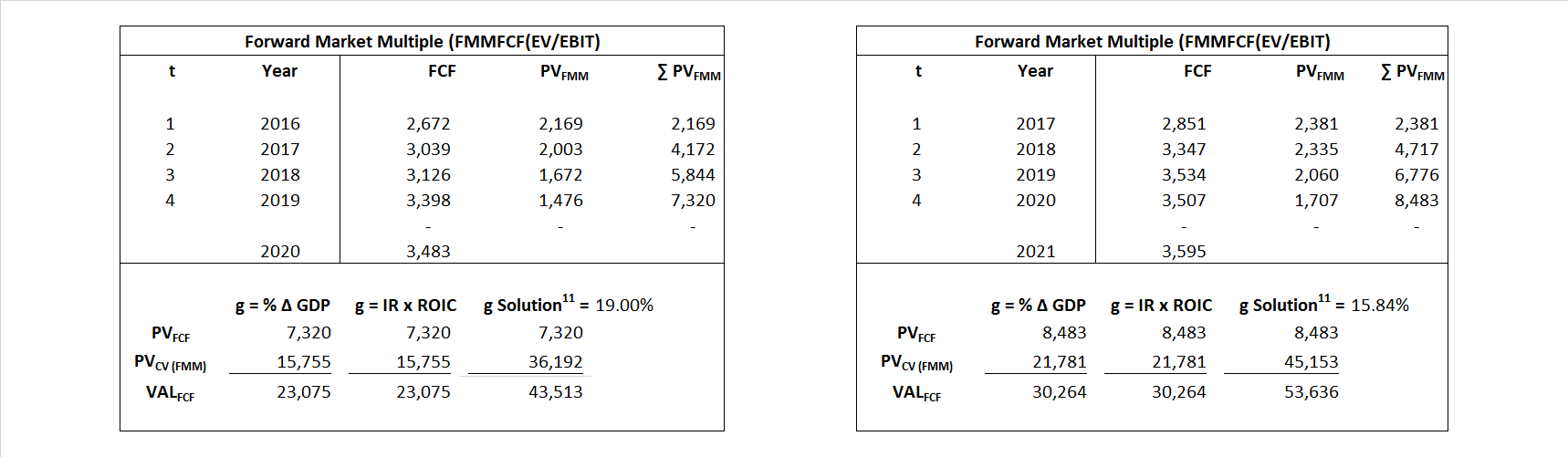# General Dynamics

## Analyst Listing

The following analysts provide coverage for the subject firm as of May 2016:

 Broker Analyst Analyst Email Deutsche Bank Research Myles Walton myles.walton@db.com Wolfe Research Hunter K. Keay hkeay@wolferesearch.com Credit Suisse Robert Spingarn robert.spingarn@credit-suisse.com Guggenheim Securities Roman Schweizer roman.schweizer@guggenheimpartners.com RBC Capital Markets Robert Stallard robert.stallard@rbccm.com CRT Capital Group Peter Arment peter.arment@bernstein.com Bernstein Research Douglas S. Harned douglas.harned@bernstein.com Cowen & Company Cai von Rumohr cai.von-rumohr@cowen.com Wells Fargo Securities Sam J. Pearlstein sam.pearlstein@wellsfargo.com Stifel Nicolaus Joseph W. DeNardi denardij@stifel.com Jefferies Howard A. Rubel hrubel@jefferies.com Buckingham Research Richard Safran rsafran@buckresearch.com

## Primary Input Data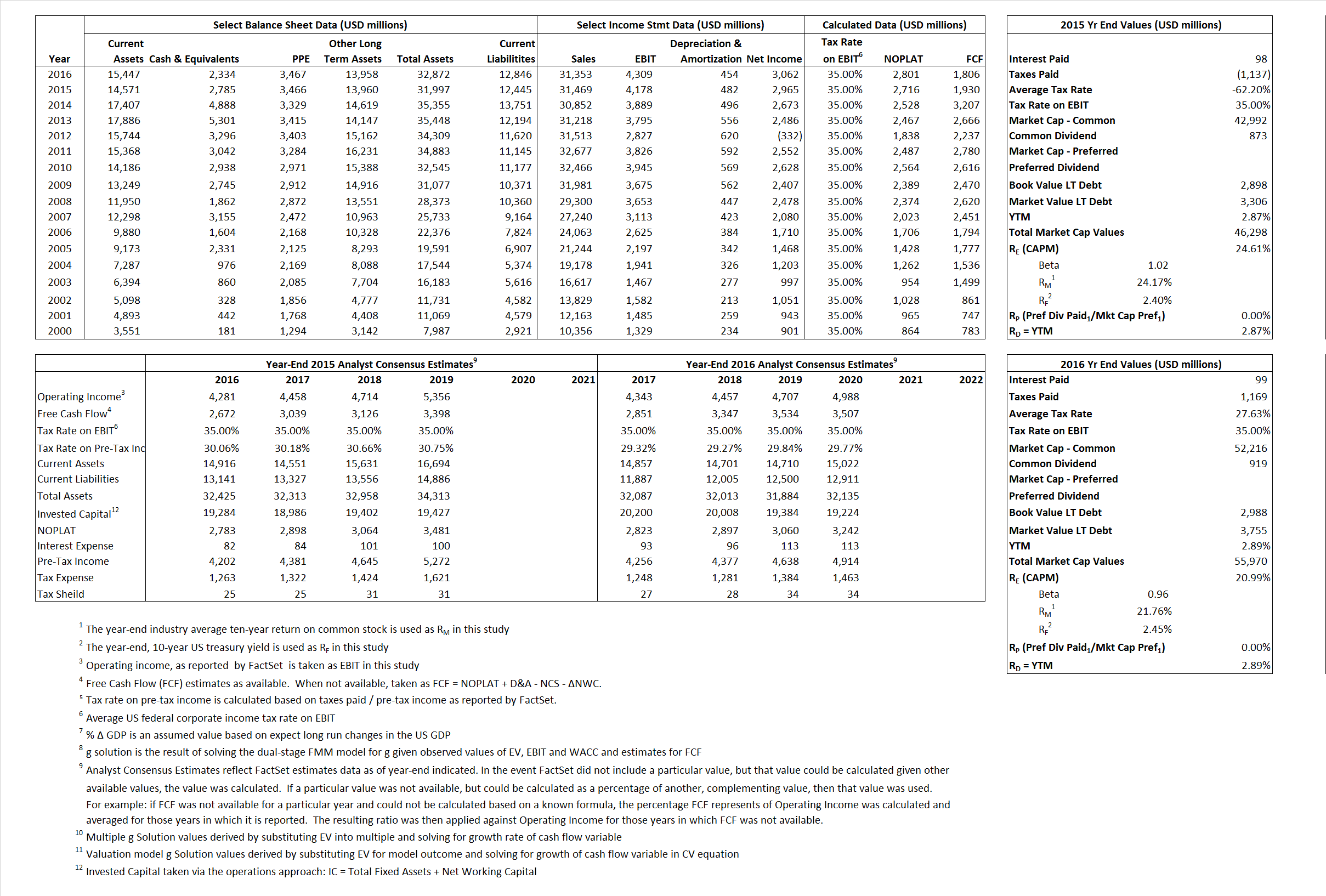## Derived Input Data

### Equational Form

Net Operating Profit Less Adjusted Taxes NOPLAT   2,716  2,801$NOPLAT\, =\, EBIT\, x\, (1 \,-\, Avg \,\,Tax\,\, Rate\,\, on\,\, EBIT)$
Free Cash Flow FCF  1,930  1,806$FCF\,=NOPLAT\,+\,Non-Cash\,Expenses-\Delta NWC\,-\,NCS$
Tax Shield TS  (61)  27$TS\,=\,Interest\,\,Paid\,\,x\,\, Avg \,\,Tax\,\,Rate\,\, on\,\, Pre-Tax\,\, Income$
Invested Capital IC  19,552  20,026$IC\,=\,Fixed\,\,Operating\,\,Assets\,\,+\,\,Net\,\, Working\,\, Capital$
Return on Invested Capital ROIC 13.89% 13.99%$ROIC\,=\,\frac { NOPLAT }{ IC }$
Net Investment NetInv  (1,570)  928$NetInv\,=\,{ {IC}_{1}}-{{IC}_{0}}+Depreciation$
Investment Rate IR -57.81% 33.13%$IR\,=\,\frac {NetInv}{NOPLAT}$
Weighted Average Cost of Capital WACCMarket 23.18% 19.72%$WACC\,=\,\frac { E }{ V } { R }_{ E }\,+\,\frac { P }{ V } { R }_{ P }\,+\,\frac { D }{ V } { R }_{ D }\left( 1- Avg\,\, Tax\,\,Rate\,\,on\,\,Pre-Tax\,\,Income \right)$
WACCBook  9.01% 8.24%
Enterprise value EVMarket  43,513  53,636$EV\,=\,Market\,\,Cap\,\,Equity\,+\,\,Long\,\,Term\,\,Debt\,-\,Cash$
EVBook  43,105  52,870
EV/EBIT Multiple$\frac{EV_{Market}}{EBIT}$  10.41  12.45$EV/EBIT\,=\,\frac { EV}{ EBIT}$
Long-Run Growth g = IR x ROIC
-8.03%   4.63% Long-run growth rates of the income variable  are used in the Continuing Value portion of the valuation models.
g = %$\Delta$ GDP    2.50%   2.50%

## Valuation Model Outcomes

The outcomes presented in this study are the result of original input data, derived data, and synthesized inputs and, depending on the equational form of any particular valuation model, may result in irrelevant or implausible results.  For example, in the event WACC < g, the value of this term, often found in the denominator of an equation’s continuation value term, will be expressly negative and may result in a negative overall valuation for the firm.  In the event of a WACC < g relation, the model form as applied to the subject firm offers an irrelevant outcome.

### Equational form

Key Value Driver (NOPLAT) KVD (NOPLAT)${ Value }_{ DCF/KVD }=\sum { \frac { NOPLAT_{ t } }{ { \left( 1+WACC \right) }^{ t } } +\frac { \frac { { NOPLAT }_{ 1 }\left( 1-\frac { g }{ ROIC } \right) }{ WACC-g } }{ { \left( 1+WACC \right) }^{ t } } }$Key Value Driver (FCF) KVD (FCF)${ Value }_{ DCF/KVD }=\sum { \frac { FCF_{ t } }{ { \left( 1+WACC \right) }^{ t } } +\frac { \frac { { NOPLAT }_{ 1 }\left( 1-\frac { g }{ ROIC } \right) }{ WACC-g } }{ { \left( 1+WACC \right) }^{ t } } }$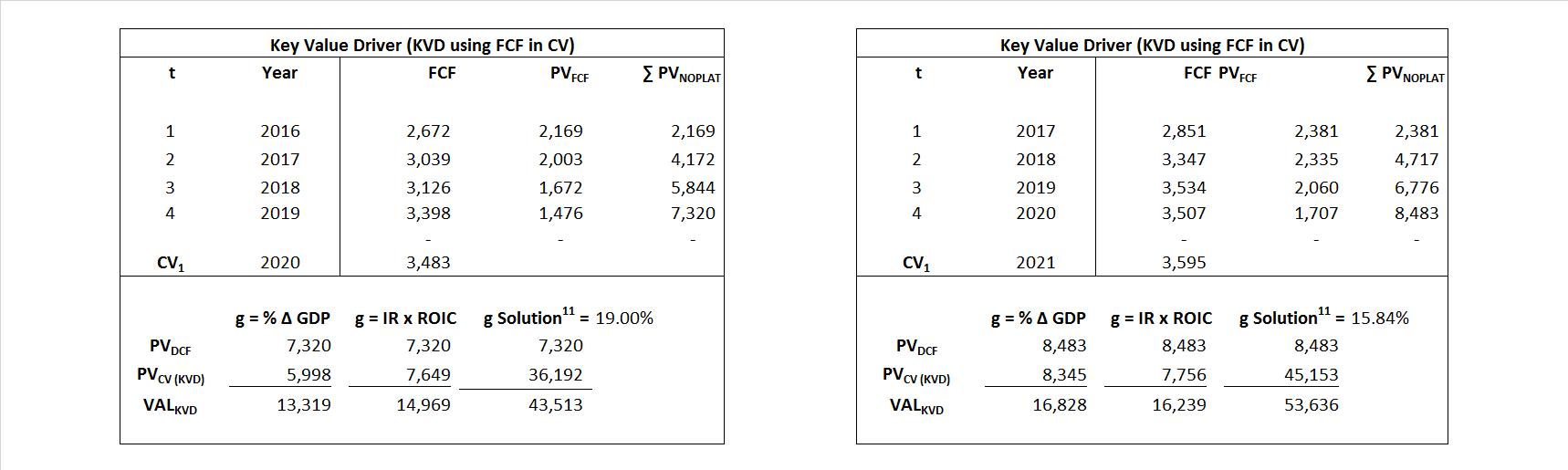Free Cash Flow FCF${ Value }_{ DCF/FCF }=\sum { \frac { FCF_{ t } }{ { \left( 1+WACC \right) }^{ t } } +\frac { \frac { { FCF }_{ 1 }}{ WACC-g } }{ { \left( 1+WACC \right) }^{ t } } }$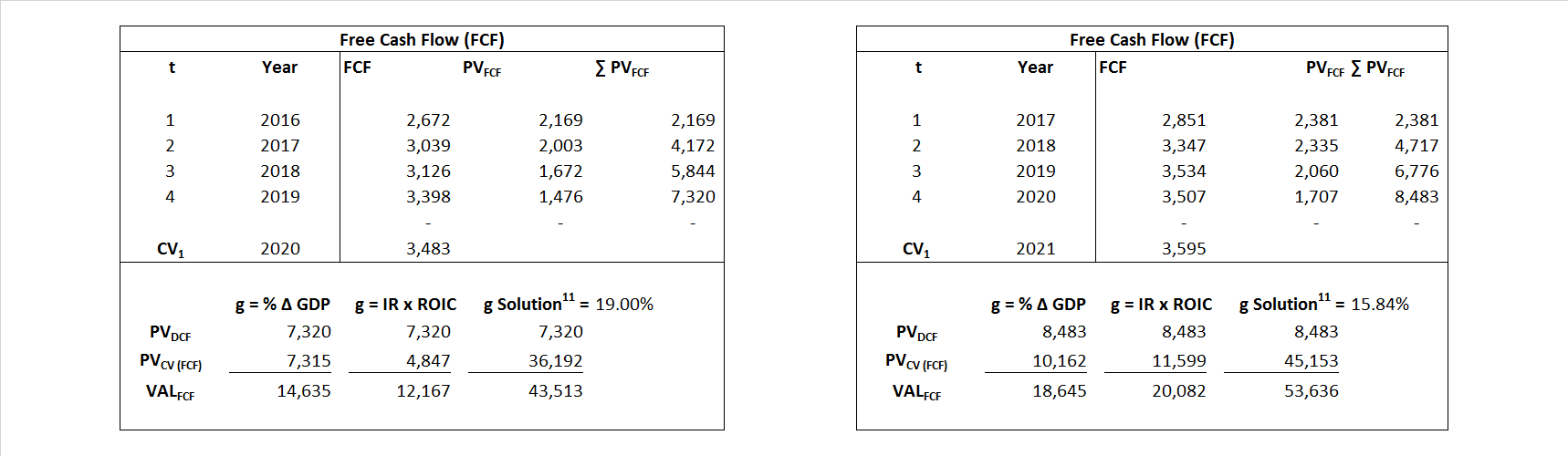Economic Profit ECON π${ Value }_{ { ECON\pi } }= I{ C }_{ 0 }+\sum { \frac { { IC }_{ t-1 }(ROI{ C }_{t}-WAC{C}_{t}) }{ { \left( 1+WACC \right) }^{ t } }+ \frac {\frac { I{C}_{0}\ x\ (ROI{C}_{1}\ -\ WAC{C}_{1}) }{ WACC-g } }{ { \left( 1+WACC \right) }^{ t } } }$Adjusted Present Value APV${ Value }_{ APV }=\sum { \frac { FCF_{ t } }{ { \left( 1+{ k }_{ u } \right) }^{ t } } +\frac { \frac { { FCF }_{ 1 }}{ { k }_{ u }-g } }{ { \left( 1+{ k }_{ u } \right) }^{ t } } } +\sum { \frac { { TS }_{ t } }{ { \left( 1+{ k }_{ tax } \right) }^{ t } } +\frac { \frac { { TS }_{ 1 }}{ { k }_{ tax }-g } }{ { \left( 1+{ k }_{ tax } \right) }^{ t } } }$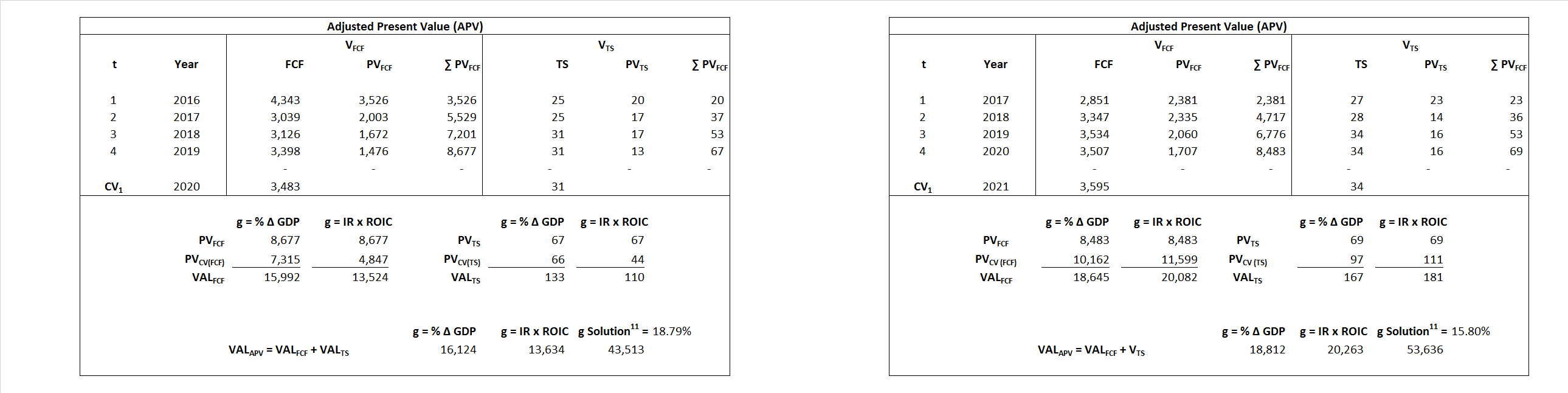Forward Market Multiple FMM${ Value }_{ DCF/FMM}=\sum { \frac { FCF_{ t } }{ { \left( 1+WACC \right) }^{ t } } +\frac { { EBIT }_{ 1 }\,{x}\,{FMM}}{ { \left( 1+WACC \right) }^{ t } } }{\,\,\,; \,\,FMM\,=\,\frac{{EV}_{t=0}}{{EBIT}_{t=0}}}$